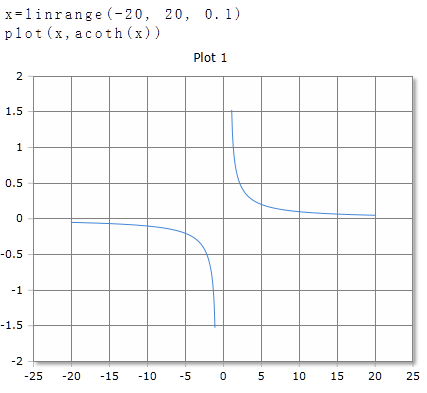# ACoth Function

Compute the inverse hyperbolic cotangent

## Description

The function $$ACoth$$ calculates the inverse hyperbolic cotangent of the argument.

$$ACoth$$ returns the angle to the hyperbolic cotangent of real or complex numbers. The argument can be a single number or a data field. For data fields, the inverse hyperbolic cotangent of each element is calculated and the results are returned in a data field of the same size.

## ACoth for real numbers

### Parameter

The argument must be less than -1 or greater than 1.

### Result

The result is given in degrees (full circle = 360 °) or radians / radians (full circle = 2 · π). The unit of measurement used is set in the toolbar with the DEG or RAD buttons or an optional parameter. The setting in the toolbar applies to the entire worksheet.

At a value of the parameter of 1 or -1, the result is ∞ (infinity). For a parameter between -1 and 1, the function returns NaN (not a number).

### Optional Parameter

Optionally, a second parameter can be specified with the keywords DEG or RAD to set the unit of measure for this function call. The specification of the parameter has priority over the global setting in the toolbar. You can use it on a worksheet different functions use different units of measurement independent of the presetting in the toolbar.

### Syntax

ACoth (value)

ACoth (value, DEG)

### Example## ACoth for complex numbers

For complex numbers, the result is always given as radians, regardless of the default setting in the toolbar. The result is also a complex number.

ACoth (re + im)

### Example

ACoth(3 + 4i)= 0.118-0.161i